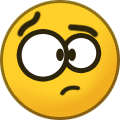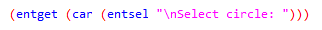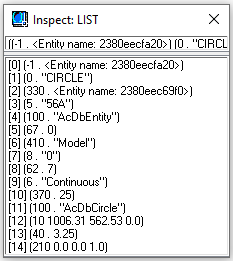# lisp coloring program## Recommended PostsHi! Hope all of u have a nice day.

I was wondering wich is the most simple way to make a lisp program that color the circles from my drawing  (with a specify radius)

Thx a lot!

##### Share on other sitesPosted (edited)

Should be what you need.

```(defun C:FOO (/ SS )
(if (setq SS (ssget '((0 . "CIRCLE") (-4 . "=") (40 . radi))))  ;change radi to the radius you want
(vl-cmdf "_Chprop" SS "" "C" "COLOR" "") ;change Color to what you want
)
)```

Edited by mhupp
•1
##### Share on other sites2 hours ago, mhupp said:

Should be what you need.

```
(defun C:FOO (/ SS )
(if (setq SS (ssget '((0 . "CIRCLE") (-4 . "=") (40 . radi))))  ;change radi to the radius you want
(vl-cmdf "_Chprop" SS "" "C" "COLOR" "") ;change Color to what you want
)
)```

and if I want the program to color the circles in the window itself, without me selecting them, what should I add?

##### Share on other sites1 hour ago, joa0009 said:

and if I want the program to color the circles in the window itself, without me selecting them, what should I add?

Don't know what you mean by window  Viewport?  This will select everything in the drawing and filter down to circles that match the radi value you put.  more info here.

```(defun C:FOO (/ SS )
(if (setq SS (ssget "_X" '((0 . "CIRCLE") (-4 . "=") (40 . radi))))  ;change radi to the radius you want
(vl-cmdf "_Chprop" SS "" "C" "COLOR" "") ;change Color to what you want
)
)```

•1
##### Share on other sitesA couple of subtle changes.

Joa0009 do you mean to hatch with solid ?

```(defun C:FOO (/ SS col rad)
(setq rad (cdr (assoc 40 (entget (car (entsel "Pick a circle "))))))
(setq col (getint "\nEnter color number "))
(if (setq SS (ssget "_X" (list (cons 0 "CIRCLE") (cons -4 "=")(cons  40  rad))))
(vl-cmdf "_Chprop" SS "" "C" col "") ;change Color to what you want
)
)```

•1
##### Share on other sitesPosted (edited)

If you mean to modify every circle in the drawing, then what @mhupp and @BIGAL suggested would work just fine. (Just with the exception to add (cons 410 (getvar 'ctab)) into the ssget filter to avoid the selection of other potential circles in other layouts as well.)

If you mean in the windows, then probably I recommend something like this:

```
(setq *circle_color* acYellow	; <-- an integer denoting AutoCAD Color Index of the circle to color
)

;; Modify every visible circle in your current viewport

(defun c:foo ( / act asc cn ex i ss)
(if (zerop (logand 8 (getvar 'undoctl))) (vla-StartUndoMark *circle_acdoc*) (setq act t))
(if (setq cn (JH:ViewCorner)
ss (ssget "_C" (car cn) (cadr cn) (list '(0 . "CIRCLE") (cons 40 *circle_radius*) (cons 410 (getvar 'ctab))))
)
(repeat (setq i (sslength ss))
(entmod
(if (setq i (1- i) ex (entget (ssname ss i)) asc (assoc 62 ex))
(subst (cons 62 *circle_color*) asc ex)
(append ex (list (cons 62 *circle_color*)))
)
)
)
)
(and (not act) (vla-EndUndoMark *circle_acdoc*))
(princ)
)

;; Modify every circle that you can "see" in your screen (basically every circle in your window.

(defun c:foo2 ( / cur i)
(vla-StartUndoMark *circle_acdoc*)
(setq cur (getvar 'cvport) i 1)
(while
(not (vl-catch-all-error-p (vl-catch-all-apply 'setvar (list 'cvport (setq i (1+ i))))))
(c:foo)
)
(setvar 'cvport cur)
(vla-EndUndoMark *circle_acdoc*)
(princ)
)

;; Viewport Corner --> Jonathan Handojo
;; Obtains the lower left and upper right coordinates of the current viewport

(defun JH:ViewCorner ( / c r s)
(setq c (trans (getvar 'viewctr) 1 2)
s (getvar 'screensize)
r (/ (getvar 'viewsize) (cadr s) 2.0)
)
(mapcar
'(lambda (x)
(trans (mapcar '(lambda (a b) (x a (* b r))) c (append s '(0.0))) 2 1)
)
(list - +)
)
)```

Edited by Jonathan Handojo
##### Share on other sitesWere still waiting for jao009, maybe a bit more.

```(setq *circle_color* (acad_colordlg 2)
*circle_radius* (cdr (assoc 40 (entget (car (entsel "\nSelect a circle")))))
)```

•1
##### Share on other sitesOn 4/30/2021 at 12:14 PM, Jonathan Handojo said:

If you mean to modify every circle in the drawing, then what @mhupp and @BIGAL suggested would work just fine. (Just with the exception to add (cons 410 (getvar 'ctab)) into the ssget filter to avoid the selection of other potential circles in other layouts as well.)

If you mean in the windows, then probably I recommend something like this:

```

(setq *circle_color* acYellow	; <-- an integer denoting AutoCAD Color Index of the circle to color
)

;; Modify every visible circle in your current viewport

(defun c:foo ( / act asc cn ex i ss)
(if (zerop (logand 8 (getvar 'undoctl))) (vla-StartUndoMark *circle_acdoc*) (setq act t))
(if (setq cn (JH:ViewCorner)
ss (ssget "_C" (car cn) (cadr cn) (list '(0 . "CIRCLE") (cons 40 *circle_radius*) (cons 410 (getvar 'ctab))))
)
(repeat (setq i (sslength ss))
(entmod
(if (setq i (1- i) ex (entget (ssname ss i)) asc (assoc 62 ex))
(subst (cons 62 *circle_color*) asc ex)
(append ex (list (cons 62 *circle_color*)))
)
)
)
)
(and (not act) (vla-EndUndoMark *circle_acdoc*))
(princ)
)

;; Modify every circle that you can "see" in your screen (basically every circle in your window.

(defun c:foo2 ( / cur i)
(vla-StartUndoMark *circle_acdoc*)
(setq cur (getvar 'cvport) i 1)
(while
(not (vl-catch-all-error-p (vl-catch-all-apply 'setvar (list 'cvport (setq i (1+ i))))))
(c:foo)
)
(setvar 'cvport cur)
(vla-EndUndoMark *circle_acdoc*)
(princ)
)

;; Viewport Corner --> Jonathan Handojo
;; Obtains the lower left and upper right coordinates of the current viewport

(defun JH:ViewCorner ( / c r s)
(setq c (trans (getvar 'viewctr) 1 2)
s (getvar 'screensize)
r (/ (getvar 'viewsize) (cadr s) 2.0)
)
(mapcar
'(lambda (x)
(trans (mapcar '(lambda (a b) (x a (* b r))) c (append s '(0.0))) 2 1)
)
(list - +)
)
)```

Hi, so this is a bit too much for me, its my first year in lisp and its hard for me to understand your program but thx for help a lot. I have a question and mabe I will solve the program by myself. What is the meaning of (setq SS (ssget '((0 . "CIRCLE") (-4 . "=") (40 . radi))). I mean why 0. "CIRCLE", -4 "="?##### Share on other sitesI always admire people who puts in the effort to learn and understand. And I will always help those type of people.

Those are the filters for the selection set. You can look up for the codes through here.

Now, let's take a circle (for example), and use the entget function on it to retrieve its properties. As an example, you can inspect this, and see the result returned:entget retrieves the properties of the object that was selected. This is an example. Now in the ssget function, you can include such filters in (except enames), so that any entities to be selected should have the properties that satisfies the criteria set within the ssget filters.

Therefore, (0 . "CIRCLE") simply means that only circles can be selected. -4 stands for a conditional operator (such and AND, OR, *, etc...).  And in the case of a circle, this will be the radius. As a first year of learning LISP, these codes may appear a bit confusing to you (because it was way too confusing for me).

You can include as many filters into ssget as you want. You can view more here.

•1
##### Share on other sites(defun C:3COLORS (/ color p1 p2 ss)
(if (and (progn (initget "Red Green Yellow" 1)
(setq color (getword "\nEnter color for the circles [Red/Green/Yellow]: "))
)
(setq p1 (getpoint "\nSpecify first point: "))
(setq p2 (getcorner p1 "\n Specify opposite corner: "))
(setq ss (ssget '((0 . "CIRCLE") (-4 . "=") (40 . 4)))
))
(progn
(command
)
)

thx a lot.. so here what function do I need to use to color my circles? (vl-cmdf "_Chprop" SS "" "C" "COLOR" "") ?

##### Share on other sites4 minutes ago, joa0009 said:

(defun C:3COLORS (/ color p1 p2 ss)
(if (and (progn (initget "Red Green Yellow" 1)
(setq color (getword "\nEnter color for the circles [Red/Green/Yellow]: "))
)
(setq p1 (getpoint "\nSpecify first point: "))
(setq p2 (getcorner p1 "\n Specify opposite corner: "))
(setq ss (ssget '((0 . "CIRCLE") (-4 . "=") (40 . 4)))
))
(progn
(command
)
)

thx a lot.. so here what function do I need to use to color my circles? (vl-cmdf "_Chprop" SS "" "C" "COLOR" "") ?

(defun C:3COLORS (/ color p1 p2 ss)
(if (and (progn (initget "Red Green Yellow" 1)
(setq color (getkword "\nEnter color for the circles [Red/Green/Yellow]: "))
)
(setq p1 (getpoint "\nSpecify first point: "))
(setq p2 (getcorner p1 "\n Specify opposite corner: "))
(setq ss (ssget '((0 . "CIRCLE") (-4 . "=") (40 . 4)))
))
(progn
(vl-cmdf "_Chprop" SS "" "C" "COLOR" "")
(prompt (strcat (itoa (sslength ss)) " Entities were changed to the color " color))
)
(prompt "\n No entities selected... ")
)
(princ)
)

##### Share on other sites8 hours ago, joa0009 said:

thx a lot.. so here what function do I need to use to color my circles? (vl-cmdf "_Chprop" SS "" "C" "COLOR" "") ?

What you posted almost works Variables don't get quotes when you call them.  This is what you need.

```(defun C:3COLORS (/ Color ss)
(initget "Red Green Yellow" 1)
(setq Color (getkword "\nEnter color for the circles [Red/Green/Yellow]: "))
(if (setq ss (ssget '((0 . "CIRCLE") (-4 . "=") (40 . 4))))
(progn
(vl-cmdf "_Chprop" SS "" "C" Color "")
(prompt (strcat "\n" (itoa (sslength ss)) " Circles Changed to Color " color)) ; this was also out of order a bit.
)
(prompt "\nNo Circles Selected...")
)
(princ)
)```

Cleaned up your code a bit. I don't know what you where trying to do with the two points but you Don't call them again.

This code is limited to circles with a radius of 4 so like @BIGAL and others have pointed out maybe use a Variable on that too.

```(defun C:3COLORS (/ Color radi ss)
(initget "Red Green Yellow" 1)
(setq Color (getkword "\nEnter color for the circles [Red/Green/Yellow]: "))
(if (setq SS (ssget (list (cons 0 "CIRCLE") (cons -4 "=") (cons 40 radi))))
(progn
(setvar 'cmdecho 0)
(vl-cmdf "_Chprop" SS "" "C" Color "")
(prompt (strcat "\n" (itoa (sslength ss)) " Circles Changed to Color " color))
(setvar 'cmdecho 1)
)
(prompt "\nNo Circles Selected...")
)
(princ)
)```

This allows you to enter a Radius if you leave it blank it will set it to 4.

Edited by mhupp

## Join the conversation

You can post now and register later. If you have an account, sign in now to post with your account.
Note: Your post will require moderator approval before it will be visible.×   Pasted as rich text.   Restore formatting

Only 75 emoji are allowed.